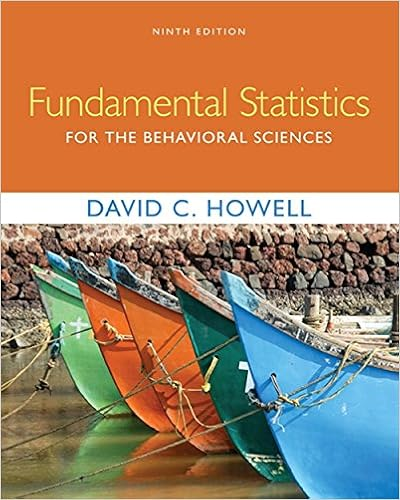# Q22the appropriate alternative hypothesis for a lower

• Test Prep
• MelvinDawson
• 24
• 92% (357) 327 out of 357 people found this document helpful

This preview shows page 23 - 24 out of 24 pages.

##### We have textbook solutions for you!
The document you are viewing contains questions related to this textbook.The document you are viewing contains questions related to this textbook.
Chapter 16 / Exercise F
Fundamental Statistics for the Behavioral Sciences
HowellExpert Verified
Q22:The appropriate alternative hypothesis for a lower tail test to determine if mean body weight of all the men who have joined a health club is less than 185 pounds would bea. HA: μ ≥ 185 lb.b. HA: μ < 185 lb. c. HA: μ = 185 lb. d. HA: μ ≠ 185 lb.
Q23: The e-mail usage for two different plants of a large company was compared at level of significance 0.05. A sample of 100 employees was selected at each plant. The mean number of e-mail messages sent per employee for one plant was 16 per week and the standard deviation was 4. For the other plant, the mean was 15 and the standard deviation was 3. For the test of equal population means versus unequal population means, the absolute value for the computed test statistic, the critical values, and the p-value respectively are:
Q24: Three samples of 10 were used to compare 3 population means. If the Sum of Squares Treatment (SST) is 350, what is the value for the Mean Square Treatment (MST)?
Q25: Which nonparametric test is analogous to a parametric two-sample t-test for means?
Q26: Which nonparametric test is analogous to a parametric t-test for differences in paired data?a. Wald-Wolfowitz testb. Wilcoxon signed rank testc. Mann-Whitney testd. Kruskal-Wallis test
##### We have textbook solutions for you!
The document you are viewing contains questions related to this textbook.The document you are viewing contains questions related to this textbook.
Chapter 16 / Exercise F
Fundamental Statistics for the Behavioral Sciences
HowellExpert Verified
Q27: Which nonparametric test is analogous to a one-factor ANOVA?
Q28: A linear regression between Y and X produced the following equation for the least squares line:= -4.3 + 2.1x Which of the following statements concerning this relationship is true?
Q29: The difference between an observed value of the dependent variable and its predicted value obtained from the regression equation is called a(n)
Q30: A regression line has been found and the statistician wants to know if the line has a slope. What is the appropriate null hypothesis to test?a. H0: β1 = 0.b. H0: β1 > 0.c. H0: β1 < 0.d. H0: β1 ≠ 0.
•••